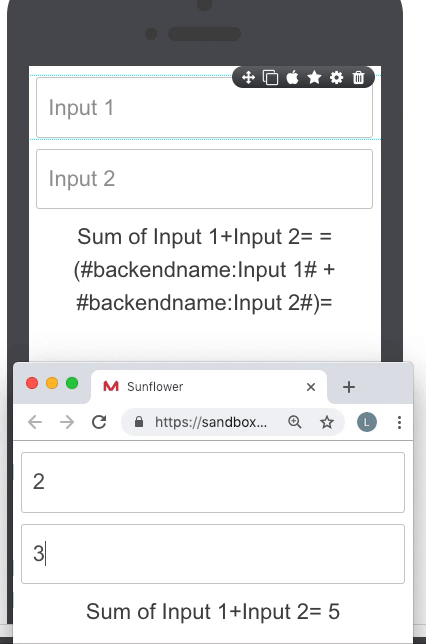##### Page tree
Go to start of banner

# Using Math Formulas

You are viewing an old version of this page. View the current version.

Version 2

Formulas are used to perform mathematical operations within app. It works at the FRONT of you app. Mobsted platform supports all arithmetical math functions and all MathJS formulas.

### Where Math is available:

You can use Math formulas AT ANY ELEMENT on the platform. For example, on screen, in values, backend names, variables, conditions to hide/show, in actions on elements,

### Referencing data in Math

You can include Hashtags references to perform calculations with any data from any part of platform and build math expressions with it.

Example:

=( #hashtag1# + #hashtag2# )=

OR

=( 3 + #hashtag2# )=

Use case:

In the example below we can see that the sum of two fields is dynamically updated when we enter the new value to the one of the inputs.### Examples of Available Math Formulas

The rest can be found here - https://mathjs.org/docs/index.html

 Action Example SUM / Multiply =( #hashtag1# + #hashtag2# )= / =( 5 * 2 )= Subtract / Divide =( #hashtag1# - #hashtag2# )= / =( 6 / 2 )= Modulus =( #hashtag1# % #hashtag2# )= Exponentiation =( #hashtag1# - #hashtag2# )= ^ =( #hashtag1# / #hashtag2# )= Square root =( sqrt(#hashtag1#) )= Factorial =( #hashtag1#! )=

You can also check the set up of some of the above functions on screen - 4 Math expressions & operations in Demo App in your profile.

• Page:
• Page:
• Page:
• Page:
• Page:

• No labels